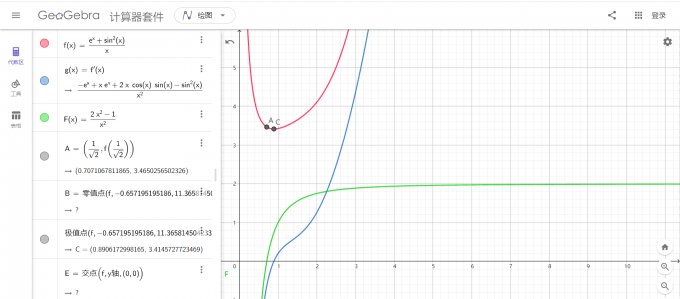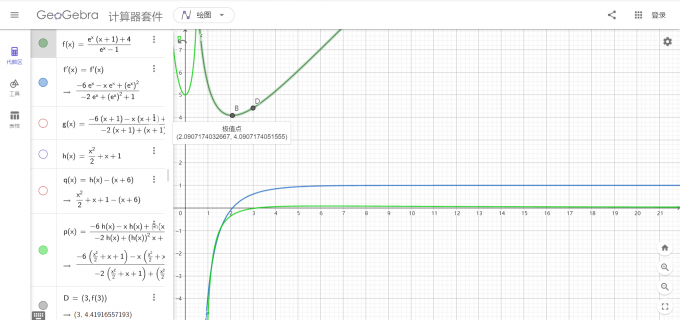## 前置知识

### 泰勒展开公式（麦克劳林级数）

$$f(x) = \sum\limits_{i=0}^{\infty} \frac{f^{(i)}(0)}{i!} x^i$$

### 常见替换

$e^x \geq x+1$ $e^x \geq \frac{x^2}{2} + x + 1$

$\ln(x) \leq x - 1$ $\ln(1+x) = x - \frac{x^2}{2}$

$\sin(x) \leq x$ $\sin(x) \geq x - \frac{x^3}{3}$

$\cos(x) \geq 1 - \frac{x^2}{2}$

## 例题1：长郡中学 2022 年高三二模第 22 题

### 题面

（1）设曲线 $y=f(x)$ 在点 $(0,f(0))$ 的切线为 $l$ ，若 $l$ 与两坐标轴所围成的三角形的面积为 $\frac{1}{2}$ ，求实数 $a$ 的值

（2）若 $a \in N^{+}$，当 $x \geq 0$ 时，$f(x) \geq 0$ 恒成立，求 $a$ 的最大值。

### 分析

#### 第二问## 例题2：长郡 2020 级高水节实验班导数专题第 3 题

### 题面

（1）当 $a=4$ 时，求 $f(x)$ 的单调区间。

（2）若不等式 $f(x) \leq (x-2)^2$ 对 $\forall x \in (0,+\infty)$ 恒成立，求实数 $a$ 的最大整数值。

### 分析

#### 采用三阶麦克劳林级数## 注意事项

1. 最好说明在零点左边导函数小于 $0$ （即零点为变号零点）
2. 检查零点是否唯一。若不唯一，有可能两个都是去极小值的零点，此时需要比较两个极小值的更小值。